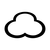# Celebrating 10 Years!May 23, 2022 -

# Fancy Math In Swift

Like Objective-C before it, Swift has access to the Accelerate framework. This is a framework made to use a computer's video card for what it does best: doing math with a bunch of floating point numbers. Commonly this is used to do all the math necessary for 3D graphics and such, but here's another handy use: signal processing.

Music, or any audio really, can be analyzed by looking at the frequency of the vibrations that make the sound we hear. For example, instruments are often tuned to the musical note A, or a frequency of 440hz. If we took an arbitrary sound file and did the math to determine the frequencies present, we could programatically determine if that note A was present. Here is how to do that kind of math:

``````import Accelerate

let bufferData = UnsafeBufferPointer<Float>(buffer)
let signal: [Float] = bufferData.map({ \$0 })
``````

First we need to get an array of floating point values. Depending on how you're accessing the audio file (is it local? is it streaming? is it from the microphone?), there are several ways to obtain this. Perhaps a subject for another article.

``````let n = vDSP_Length(44100)
let log2n = vDSP_Length(log2(Float(n)))
guard let fftSetUp = vDSP.FFT(log2n: log2n, radix: .radix2, ofType: DSPSplitComplex.self) else {
fatalError("Failed to setup FFT")
}
``````

Here we need to setup the FFT. FFT stands for "fast fourier transform", which is the mathematical process we'll use to detect frequencies in the audio signal. An important detail here is our value for `n`. Audio is typically recorded with a sampling frequency of 44.1khz, this `n` value needs to be that same number. If you don't want to get into the calculus that does this, just make sure this `n` matches the sample rate of the audio you're analyzing.

Next we have some more setup to do before we can run the actual math. Because of the way swift works with pointers and the way the framework uses pointers this gets a little weird, but at least this part won't change regardless of what kind of audio you're analyzing.

``````
let halfN = Int(n/2)
var forwardInputReal = [Float](repeating: 0, count: halfN)
var forwardInputImag = [Float](repeating: 0, count: halfN)
var forwardOutputReal = [Float](repeating: 0, count: halfN)
var forwardOutputImag = [Float](repeating: 0, count: halfN)
forwardInputReal.withUnsafeMutableBufferPointer { forwardInputRealPtr in
forwardInputImag.withUnsafeMutableBufferPointer { forwardInputImagPtr in
forwardOutputReal.withUnsafeMutableBufferPointer { forwardOutputRealPtr in
forwardOutputImag.withUnsafeMutableBufferPointer { forwardOutputImagPtr in

// Create a `DSPSplitComplex` to contain the signal.
var forwardInput = DSPSplitComplex(realp: forwardInputRealPtr.baseAddress!, imagp: forwardInputImagPtr.baseAddress!)

// Convert the real values in `signal` to complex numbers.
signal.withUnsafeBytes {
vDSP.convert(interleavedComplexVector: [DSPComplex](\$0.bindMemory(to: DSPComplex.self)), toSplitComplexVector: &forwardInput)
}

// Create a `DSPSplitComplex` to receive the FFT result.
var forwardOutput = DSPSplitComplex(realp: forwardOutputRealPtr.baseAddress!, imagp: forwardOutputImagPtr.baseAddress!)

// Perform the forward FFT.
fftSetUp.forward(input: forwardInput, output: &forwardOutput)
}
}
}
}

var autospectrum = [Float](unsafeUninitializedCapacity: halfN) {
autospectrumBuffer, initializedCount in

// The `vDSP_zaspec` function accumulates its output. Clear the
// uninitialized `autospectrumBuffer` before computing the spectrum.
vDSP.clear(&autospectrumBuffer)

forwardOutputReal.withUnsafeMutableBufferPointer { forwardOutputRealPtr in
forwardOutputImag.withUnsafeMutableBufferPointer { forwardOutputImagPtr in

var frequencyDomain = DSPSplitComplex(realp: forwardOutputRealPtr.baseAddress!, imagp: forwardOutputImagPtr.baseAddress!)
The final result sits in our variable `autospectrum` here at the end. It is an array of floats where each value represents the amount of signal (or power) detected at a given frequency. For example, if our source audio was a pure A note for tuning, we would see 0's in every position in the array except for the value at index 440. Keep in mind most tones aren't pure singular frequencies, so you may see smaller values in other spaces.